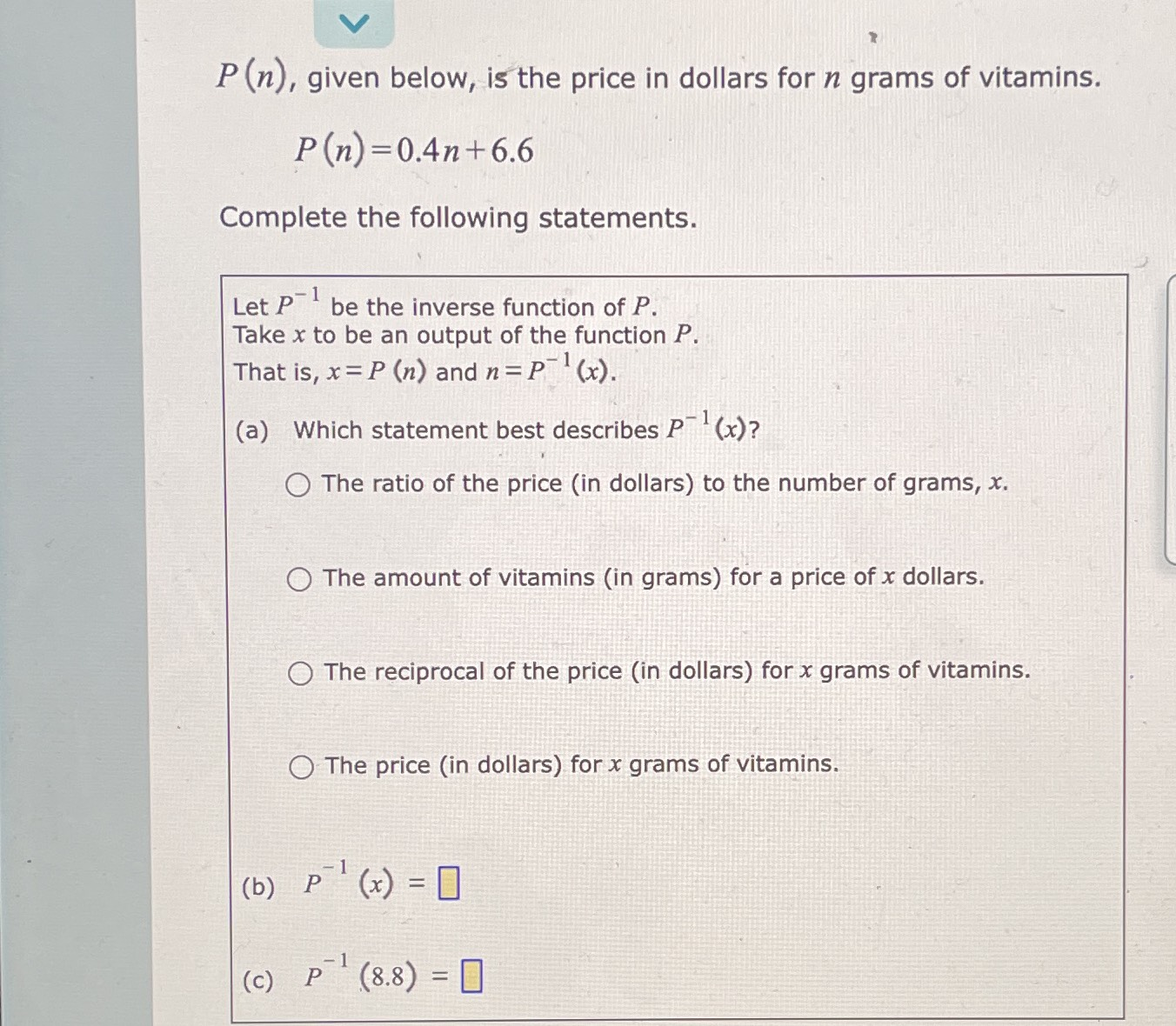### Still have math questions?

Algebra
Question$$P ( n )$$ , given below, is the price in dollars for $$n$$ grams of vitamins.

$$P ( n ) = 0.4 n + 6.6$$

Complete the following statements.

Let $$P ^ { - 1 }$$ be the inverse function of $$P$$ . Take $$x$$ to be an output of the function $$P$$ .

That is, $$x = P ( n )$$ and $$n = P ^ { - 1 } ( x )$$ .

(a) Which statement best describes $$P ^ { - 1 } ( x )$$ ?

The ratio of the price (in dollars) to the number of grams, $$x$$ .

The amount of vitamins (in grams) for a price of $$x$$ dollars.

The reciprocal of the price (in dollars) for $$x$$ grams of vitamins.

The price (in dollars) for $$x$$ grams of vitamins.

(b) $$P ^ { - 1 } ( x ) = \square$$

(c) $$P ^ { - 1 } ( 8.8 ) = \square$$

(b) $$P^ { - 1} ( x) = \frac { 5x- 33} { 2}$$
(c) $$P^ { - 1} ( 8.8) = 5.5$$